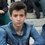# Sum of divisors

For each positive integer n, let $\sigma(n)$ denote the sum of all its positive divisors (including 1 and itself).

Determine all positive integers $n > 1$, such that there doesn't exist any positive integer m that satisfies: $n < m^2 \leq \sigma(n)$.Note by Tomás Carvalho
5 years, 9 months ago

This discussion board is a place to discuss our Daily Challenges and the math and science related to those challenges. Explanations are more than just a solution — they should explain the steps and thinking strategies that you used to obtain the solution. Comments should further the discussion of math and science.

When posting on Brilliant:

• Use the emojis to react to an explanation, whether you're congratulating a job well done , or just really confused .
• Ask specific questions about the challenge or the steps in somebody's explanation. Well-posed questions can add a lot to the discussion, but posting "I don't understand!" doesn't help anyone.
• Try to contribute something new to the discussion, whether it is an extension, generalization or other idea related to the challenge.

MarkdownAppears as
*italics* or _italics_ italics
**bold** or __bold__ bold
- bulleted- list
• bulleted
• list
1. numbered2. list
1. numbered
2. list
Note: you must add a full line of space before and after lists for them to show up correctly
paragraph 1paragraph 2

paragraph 1

paragraph 2

[example link](https://brilliant.org)example link
> This is a quote
This is a quote
    # I indented these lines
# 4 spaces, and now they show
# up as a code block.

print "hello world"
# I indented these lines
# 4 spaces, and now they show
# up as a code block.

print "hello world"
MathAppears as
Remember to wrap math in $$ ... $$ or $ ... $ to ensure proper formatting.
2 \times 3 $2 \times 3$
2^{34} $2^{34}$
a_{i-1} $a_{i-1}$
\frac{2}{3} $\frac{2}{3}$
\sqrt{2} $\sqrt{2}$
\sum_{i=1}^3 $\sum_{i=1}^3$
\sin \theta $\sin \theta$
\boxed{123} $\boxed{123}$

Sort by:

(23)<(24)= (an>af) can you help me to solve that problems? thank you

- 4 years, 4 months ago

wow i like math can you teach me?

- 4 years, 4 months ago

For each positive integer $n$, let $\sigma(n)$ be the sum of its divisors (including 1 and $n$). Determine all positive integers $n > 1$ such that there doesn't exist any positive integer $m$ that satisfies $n < m^2 \le \sigma(n)$.

We claim the only such numbers are the prime numbers and the square of prime numbers, excluding 3.

First, if $p$ is a prime number, then $\sigma(p) = p+1$ and $\sigma(p^2) = p^2+p+1$. Then any such $m$ satisfying $p < m^2 \le \sigma(p)$ must satisfies $m^2 = p+1$, or $p = m^2 - 1$. Except for $p = 3$, this is a composite number (it has factors $m-1, m+1$). Any such $m$ satisfying $p^2 < m^2 \le \sigma(p)$ must satisfy $m \ge p+1$ because $m^2 > p^2$, but this leads to $m^2 = p^2+2p+1 > p^2+p+1 = \sigma(p)$, contradiction.

Now, $n = 3$ is a special case. Otherwise, if $n$ is not a prime number or the square of one, then $n$ has at least two additional distinct factors $a,b$ which multiply to $n$. By AM-GM inequality, $\frac{a+b}{2} \ge \sqrt{ab}$ or $a+b \ge 2\sqrt{n}$; since $a \neq b$, equality is not achieved, so $a+b > 2\sqrt{n}$. Thus $\sigma(n) \ge 1+a+b+n > n+2\sqrt{n}+1 = (\sqrt{n}+1)^2$. But there must be a square number $m^2$ between $(\sqrt{n}^2, (\sqrt{n}+1)^2]$; this satisfies $n = \sqrt{n}^2 < m^2 \le (\sqrt{n}+1)^2 \le \sigma(n)$, contradiction.

- 5 years, 9 months ago

@Ivan Koswara Oh and if you like these problems, here's a harder one (I have literally made no progress on it): Let n be a positive integer. We mean to paint every integer from 1 to n in blue or red. We say that the coloration is "balanced" if the following property is verified: if a and b are integers between 1 and n, painted in different colors, then, if a+b is also an integer between 1 and n, a+b is painted in blue, and if ab is also an integer between 1 and n, ab is painted in red. Let N be the number of "balanced" colorations of the integers from 1 to n. Let P be the number of prime numbers p with the property that the largest integer smaller or equal to n/p is a power of p with a postive integer exponent. Prove that: N=1+n+P.

- 5 years, 9 months ago

@IvaThank you very much, it's a very nice solution. I had been able to prove it doesn't work for primes except for 3 and for squares of primes, but i just couldn't prove that it works for every other number. Thanks! ;)

- 5 years, 9 months ago

@Ivan Koswara wow! nice solution ... how did yo think of tht ... Never seen such a problem .... Could yo provide links to such problems ..... Thanks :)

- 5 years, 9 months ago

It's an Olympiad Problem, I don't know the link to this one in particular but if you search Math Olympiads online I'm sure you'll find many challenging problems. (If you're looking for even harder ones search IMO problems)

- 5 years, 9 months ago

@Aareyan Manzoor Can you help me solve these two other problems I've posted? Thank you.

- 5 years, 9 months ago